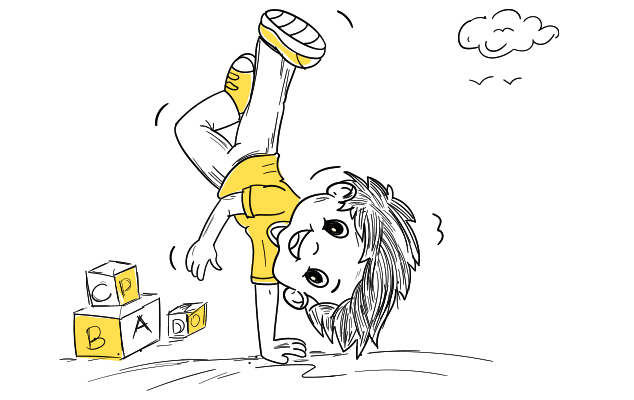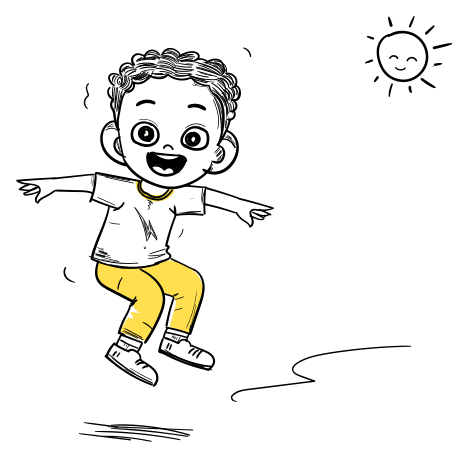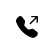LMonk Solutions
info@lmonk.com

# Dyscalculia

###### Dyscalculic Statistician## What is Dyscalculia?

Dyscalculia is a learning disability that makes it difficult for a child to understand math-related concepts, and basic mathematical skills like addition, subtraction, word problems, etc.

3% to 14% of children are reported to have maths difficulties according to a recent study, although the actual rate is far greater. Consequently, if a school has around 2000 students, roughly 250–300 of them will experience difficulties with their mathematics abilities. Imagine the unattainable figure, which may be much higher than this recognized figure.

The negative link between a child’s maths capability and maths anxiety is one of the devastating consequences of neglecting a maths learning disability. It is hypothesized that there is an inverse relationship between maths aptitude and maths anxiety that may get stronger with age and/or education. That is to say, the rate of development has risen.### Symptoms

•          Struggle to count forward or backward numbers,
•          Reversals or mirror imaging of numbers (12 for 21 or P for 9, etc.)
•         Difficulty in understanding basic math concepts like addition, subtraction, multiplication, and division
•          Confusion in identifying the mathematical symbols (+/x, and -/÷),
•          Comprehend word problems etc.## For more details,

Call us straight away. We’ll be glad to hear from you.#### +91 902 030 4535